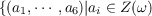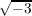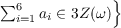#### Vol. 36, No. 2, 1971

 Download this articleFor screen For printingRecent Issues Vol. 323: 1  2 Vol. 322: 1  2 Vol. 321: 1  2 Vol. 320: 1  2 Vol. 319: 1  2 Vol. 318: 1  2 Vol. 317: 1  2 Vol. 316: 1  2Online Archive Volume: Issue:The Journal Subscriptions Editorial Board Officers Contacts Submission Guidelines Submission Form Policies for Authors ISSN: 1945-5844 (e-only) ISSN: 0030-8730 (print) Special Issues Author Index To Appear Other MSP Journals
On a six-dimensional projective representation of PSU4(3)

### John Hathway Lindsey, II

Vol. 36 (1971), No. 2, 407–425
##### Abstract

In the course of an investigation ofsix-dimensional complex linear groups, it was discovered that a central extension of Z6 by PSU4(3) has a representation of degree six. In fact, this representation has as its image the unimodular subgroup X(G) of index 2 of the following 6-dimensional matrix group:all 6 by 6 permutaion matrices; all unimodular diagonal matrices of order 3; I6 Q∕3 where Q has all its entries equal to one. This matrix group leaves the following lattice invariant:where throughout this paper ω is a primitive third root of unity; ai ajZ(ω) for all i,j;. The generators of the matrix group are similar to the following generators for an 8-dimensional complex linear group with Jordan-Holder constituents Z2, the nontrivial simple constituent of 08(2),Z2;all 8 by 8 permutation matrices, all unimodular diagonal matrices of order 2, I8 P∕4 where P has all entries equal to 1.

The projective representation of PSU4(3) can be used to construct a 12-dimensional representation Y (H), a central extension of Z6 by the Suzuki group, which leads to the known 24-dimensional projective representation of the Conway group. In fact, H has a subgroup K isomorphic to a central extension of (Z6 × Z3) by PSU4(3). Also, Y |H has two six-dimensional constituenls coming from the above matrix group where the constiluents are related by an outer automorphism of PSU4(3) which does not lift to the central extension of Z6 by PSU4(3) with the six-dimensional representation. We obtain two commuting automorphisms, α and β respectively, of G from I6 Q∕3 and complex conjugation. For PSU4(3), the outer automorphism group is dihedral of order eight with its center corresponding to complex conjugation of X(G). The entire automorphism group lifls to K. We may take the center of K to be a,b,c with a and b of order 3 and c of order 2, with GK∕b, and with α(a) = a, α(b) = b1, β(a) = a1, β(b) = b1. We can also find an automorphism γ of K with γ(a) = b and γ(b) = a. We give the character table of K giving only one representative of each family of algebraically conjugate characters and classes. Irrational characters and classes are underlined. Only one class in each coset of Z(K) is represented by the character tables. The characters in the table U4(3) give the characters with Z(K) in the kernel. The succeeding five character tables in order give the following linear characters, respectively, on Z(K) : 𝜃(a) = 𝜃(b) = 1,0(c) = 1; 𝜃(a) = ω, 𝜃(b) = 𝜃(c) = 1; 𝜃(a) = ω1, 𝜃(b) = 1, 𝜃(c) = 1; 𝜃(a) = 𝜃(b) = ω, 𝜃(c) = 1; 𝜃(a) = 𝜃(b) = ω, 𝜃(c) = 1. The characters with other actions are obtained by applying elements of the outer automorphism group. The automorphism α transposes π7 with π71; and N1 with N11 in the character tables. The automorphism β transposes N1 with N11; and N2 with N21. The automorphism γ transposes T1 with T2; JT1 with JT2; N1 with N2; N11 with N21; and possibly π7 with π71. As SU4(3)Ω(ZSU4(3)) has the centralizer of some central involution isomorphic to the centralizer of some central involution J in G, presumably SU4(3)Ω1(ZSU4(3))G∕03(Z(G)).

Primary: 20.70
##### Milestones